## 11.4.2 Continuous-Time Information Spaces

Now assume that there is a continuum of stages. Most of the components of Section 11.4.1 remain the same. The spaces,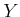,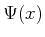,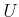, and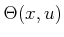remain the same. The sensor mapping also remains the same. The main difference occurs in the state transition equation because the effect of nature must be expressed in terms of velocities. This was already introduced in Section 10.6. In that context, there was only uncertainty in predictability. In the current context there may be uncertainties in both predictability and in sensing the current state.

For the discrete-stage case, the history I-states were based on action and observation sequences. For the continuous-time case, the history instead becomes a function of time. As defined in Section 7.1.1, let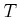denote a time interval, which may be bounded or unbounded. Let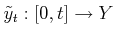be called the observation history up to time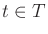. Similarly, let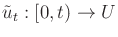and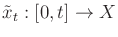be called the action history and state history, respectively, up to time.

Thus, the three kinds of sensor mappings in the continuous-time case are as follows:

1. A state-sensor mapping is expressed as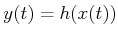, in which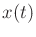and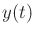are the state and observation, respectively, at time.
2. A state-nature mapping is expressed as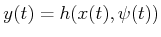, which implies that nature chooses some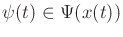for each.
3. A history-based sensor mapping, which could depend on all of the states obtained so far. Thus, it depends on the entire function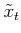. This could be denoted as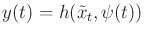if nature can also interfere with the observation.

If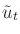and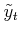are combined with the initial condition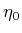, the history I-state at time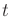is obtained as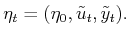(11.53)

The history I-space at timeis the set of all possible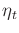and is denoted as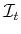. Note thatis a space of functions because each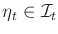is a function of time. Recall that in the discrete-stage case, every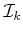was combined into a single history I-space,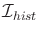, using (11.18) or (11.19). The continuous-time analog is obtained as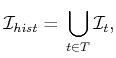(11.54)

which is an irregular collection of functions because they have different domains; this irregularity also occurred in the discrete-stage case, in whichwas composed of sequences of varying lengths.

A continuous-time version of the cost functional in Formulation 11.1 can be given to evaluate the execution of a plan. Let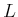denote a cost functional that may be applied to any state-action history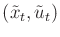to yield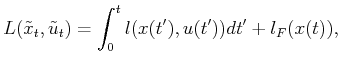(11.55)

in which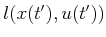is the instantaneous cost and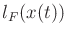is a final cost.

Steven M LaValle 2020-08-14